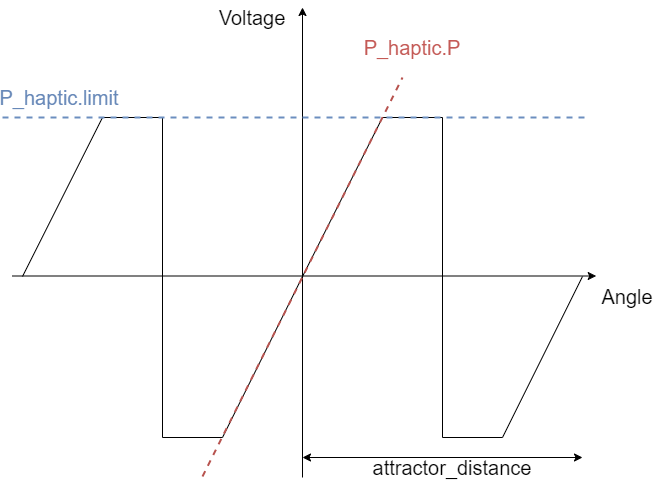# Haptic textures

I’d like to implement a smart input lever with detents. I did a quick test setting the angle target based on the shaft angle, when it is 75% to the target the target moves to the next detent. This works but it doesn’t feel right. Anyone have ideas on how to adjust the torque curve in real time so it feels like a real clicky detent?

I take it you are mostly tweaking voltage_limit (which approximated to torque) and the P term of the angle PID.

I like the term haptic texture! Did you invent that?

i’ve had not much success. It seems you need to re- init() to get the voltage_limit to update and that causes a delay.

here is what i have so far

Hey @schoch
This is a very interesting application and I would be very happy to see your results.

I would not use the `voltgae_limit` for this kind of application. Here is a very simple code that works pretty well for me. I am not sure if it is what you were searching for:

``````#include <SimpleFOC.h>

// BLDC motor & driver instance
BLDCMotor motor = BLDCMotor(11);
BLDCDriver3PWM driver = BLDCDriver3PWM(5, 10, 6, 7);

// encoder instance
Encoder encoder = Encoder(2, 3, 500);

// Interrupt routine intialisation
// channel A and B callbacks
void doA(){encoder.handleA();}
void doB(){encoder.handleB();}

void setup() {

// initialize encoder sensor hardware
encoder.init();
encoder.enableInterrupts(doA, doB);
// link the motor to the sensor

// driver config
// power supply voltage [V]
driver.voltage_power_supply = 12;
driver.init();

// set motion control loop to be used
motor.controller = ControlType::voltage;

// use monitoring with serial
Serial.begin(115200);
// comment out if not needed
motor.useMonitoring(Serial);

// initialize motor
motor.init();
// align sensor and start FOC
motor.initFOC();

_delay(1000);
}

// haptic attraction controller - only Proportional
PIDController P_haptic{.P=10,.I=0,.D=0,.output_ramp=100000,.limit=12};
// attractor angle variable
float attract_angle = 0;
// distance between attraction points
float attractor_distance = 45*_PI/180.0; // dimp each 45 degrees

float findAttractor(float current_angle){
return round(current_angle/attractor_distance)*attractor_distance;
}

void loop() {

// main FOC algorithm function
motor.loopFOC();

// Motion control function
motor.move(P_haptic(attract_angle - motor.shaft_angle));

// calculate the attractor
attract_angle = findAttractor(motor.shaft_angle);
}
``````

I would suggest you to create your own control loop using the `PIDController` class and you in it use only the proportional value `P`. This will give you a linear feedback, which seems good to meThe haptic texture will be something like this:It is defined by the slope `P_haptic.P` and the voltage limit in the `P_haptic.limit`. And in the case of my code the switching in between the attraction points is done exactly in the midle of the two `attractor_distance/2`.
This is a very simple code though. I am sure you can do it much betterWow, thanks for the detailed help!!!

Below is a quick video I did which explains the specific application (lathe contoller). I’ll work on incorporating your feedback and do a follow up sharing my code. This was inspired by a project I saw on hackaday called “turn by wire” https://rutian.github.io/projects/TBW/

2 Likes

an interesting application doable with simpleFOC

1 Like

For anyone following along: Here is a 4 position virtual toggle. There is a “click” feel when you move from center to the right or left 30 degrees. Moving to the right 60 degrees you feel a 2nd click and the torque increases.

First thing was to define 3 PIDController instances

``````// attractor angle variable
float attract_angle = 0;
// distance between attraction points
int distance_degrees = 30;
float attractor_distance = distance_degrees * _PI / 180.0;
// track the current "detent"
int detent_num = 0;

// controllers
PIDController strongPID{.P=40,.I=0,.D=0,.output_ramp=100000,.limit=12};
PIDController midPID{.P=10,.I=0,.D=0,.output_ramp=100000,.limit=6};
PIDController weakPID{.P=10,.I=0,.D=0,.output_ramp=100000,.limit=4};
...
``````

Then in the loop you can do away with the findAttractor, it always will pull to 0 degrees.

``````void loop() {
motor.loopFOC();

// Motion control function
float pid_input = 0;
switch(abs(detent_num)){
case 0:
pid_input = weakPID(attract_angle - motor.shaft_angle);
break;
case 1:
pid_input = midPID(attract_angle - motor.shaft_angle);
break;
case 2:
pid_input = strongPID(attract_angle - motor.shaft_angle);
break;
}
motor.move(pid_input);
if(motor.shaft_angle >= 2*attractor_distance){
detent_num = 2;
}else if(motor.shaft_angle >= attractor_distance){
detent_num = 1;
}else if(motor.shaft_angle <= -attractor_distance){
detent_num = -1;
}else {
detent_num = 0;
}

}
``````

pretty cool! I’m going to hook up a stepper to see if allows for a bigger torque range and better feel.

1 Like

Hello, thank you for this interesting topic! This is exactly what I’m trying to do. An indexing knob with various ratchet numbers. As a beginner, I built a prototype with a small BLDC gimbal motor, an SPI position sensor and the famous simpleFoc board and libraries (what an impressive work!). I can feel the different ratchets, but my main problem is the instability on each detent of my motor. To stabilize it, the only solution I’ve found is to add friction on the shaft. Even with low proportional coefficient, the motor seems to “over-react”. Do you have any good idea to help me to have a more stable system? Thank you.

Hey @jeanda25,
You could play a bit with the derivative coefficient, it should in theory be similar to the viscose friction. (proportional) to the velocity of the movement.
It is can easily become unstable though. So you might need to filter the position a bit